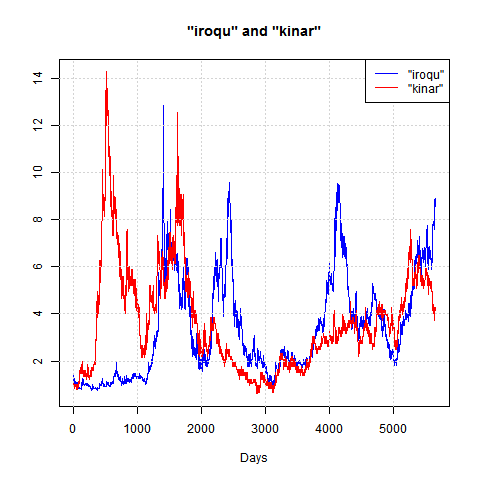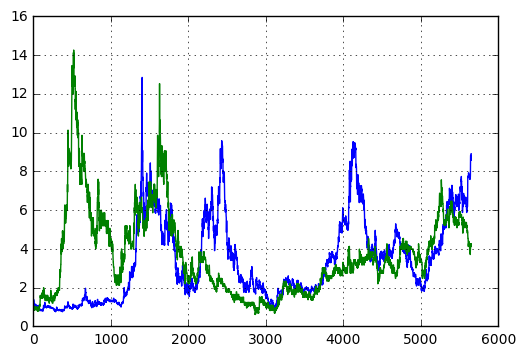## Monday, February 20, 2017

### Rebooting with Python and Jupyter

This blog has been inactive for a long time for essentially two reasons:

• I was not very happy with the quality of the results
• The source code was not showing very nicely
• It was difficult to get a nice display, including for pictures and mathematical expressions
• I started to use Python almost exclusively
• R is a nice language, but it is not a general purpose language, some tasks are hard in R compared to Python
• At the other hand, Python has steadily improved in the area of data processing, with pandas providing something equivalent to the R dataframe
But now, there is a good way to solve both problems, Jupyter notebooks combined with the ability to directly include HTML in a post.  So it is time for a reboot.

Jupyter was originally know as iPython but has evolved to support many programming languages, including R.  This allows now to develop a notebook, possibly based on multiple languages, then convert it for posting, while keeping the original notebook available for people that wants a more interactive experience.  The development process is much simpler that way that it used to be for earlier posts.

As an example, the rest of this post is this notebook converted to HTML.  Note that the notebook contains both R and Python code interacting in an almost seamless way.  How to achieve that result will be explained in later posts.

In :
%load_ext rpy2.ipython

In :
%%R -o x -o xik -o n -o pik
# figure 8.1 of Cover "Universal Portfolios"

library(logopt)
data(nyse.cover.1962.1984)
n <- nyse.cover.1962.1984
x <- coredata(nyse.cover.1962.1984)
xik <- x[,c("iroqu","kinar")]
nDays <- dim(xik)
Days <- 1:nDays
pik <- apply(xik,2,cumprod)
plot(Days, pik[,"iroqu"], col="blue", type="l",
ylim=range(pik), main = '"iroqu" and "kinar"', ylab="")
lines(Days, pik[,"kinar"], col="red")
grid()
legend("topright",c('"iroqu"','"kinar"'),
col=c("blue","red"),lty=c(1,1))In :
print(x)
print(type(x))
import matplotlib as mpl
import matplotlib.pyplot as plt
plt.ion()
plt.figure(figsize=(6,4))
plt.plot(pik)
plt.grid()

[[ 1.01515  1.02765  1.04183 ...,  1.00578  0.99697  0.99752]
[ 1.01493  1.04036  0.98905 ...,  1.00958  0.99088  1.00248]
[ 1.       0.97629  0.97786 ...,  1.       1.02761  0.99752]
...,
[ 0.99029  0.9966   0.99605 ...,  0.99216  1.00461  0.99273]
[ 0.99265  1.00683  1.      ...,  0.99209  1.02752  1.00366]
[ 0.99753  1.00339  1.01984 ...,  1.01195  1.       0.99635]]
<class 'numpy.ndarray'>